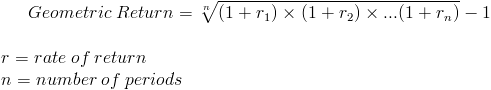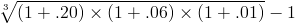# Geometric Mean ReturnThe geometric mean return formula is used to calculate the average rate per period on an investment that is compounded over multiple periods. The geometric mean return may also be referred to as the geometric average return.

## Use of the Geometric Mean Return Formula

The formula for the geometric mean return is used specifically for investments that are compounded. By contrast, a simple interest account would use the arithmetic average which is summing the rates and dividing by the number of periods.

The geometric mean return formula can also be used to break down the effective rate per period of the holding period return. The holding period return is the total return over multiple periods.

## Example of the Geometric Mean Return Formula

A simple example of the geometric mean return formula would be \$1000 in a money market account that earns 20% in year one, 6% in year two, and 1% in year three.

It would be incorrect to use the arithmetic mean of adding the rates together and dividing them by three. With this example, the arithmetic mean would be 9%, as shown by summing the rates and dividing by three. By incorrectly using this method, the ending balance of 9% per year would return a balance of \$1295.03.

Using the formula for compound interest with different rates, the ending balance after year three can be found by multiplying the balance times 1.20, 1.06, and 1.01. The ending balance after year three would be \$1284.72. Notice the differences between the ending balance with incorrectly using the arithmetic mean shown in the prior paragraph and the actual ending balance.

The equation for this example using the formula for the geometric mean return would bewhich would return 8.71%. This answer can be checked by using the compound interest formula which would return \$1284.72 as shown in the prior paragraph.

New to Finance?